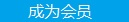您可以捐助，支持我们的公益事业。 1元 10元 50元 认证码：必填求知 文章 文库 Lib 视频 iProcess 课程 角色 咨询 工具 讲座 Modeler Code要资料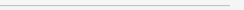订阅捐助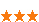1477 次浏览     评价： 好 中 差
2018-9-28

 编辑推荐: 本文来自于CSDN，介绍了CNN分类问题以及如何用matlab自带数据集做车辆检测等相关知识。

1.前言

2.配置需求

3. 可以完成的任务

1. 获取别人训练好的CNN网络

2. 迁移学习(transfer learning and fine-tune)

3. 解决分类问题(classifiy problem)

4. 解决回归问题(regression problem)

5. 物体检测(object detection)

6. 提取学习到的特征

3.1 获取别人训练好的网络

matlab2017中，可以用别人训练好的现成的网络，也可以输入caffe中的网络。目前已知的可以用的网络包括用于分类的：Alexnet, vgg16, vgg19。已经用于物体检测的，RCNN, FastRCNN, Faster RCNN。由于最近一直研究的是分类和回归问题，物体检测的CNN在过后补全。这里只举一个分类的例子。

Alexnet作为2012年ImageNet的冠军，它的提出确实影响到了CV的研究热点，人们惊奇的发现深度网络的描述能力居然这么强，虽然背后的数学原理一直没能得到完美的解决，但不妨碍它强大的能力，我们看看她在matlab中是如何做分类的。首先贴出代码：

 clear;clc;close all; %获取alexnet net = alexnet; %读照片选物体 I= imread('peppers.png'); [cropedim, rect2]=imcrop(I); cropedim=imresize(cropedim,[227 227]); figure,imshow(cropedim); % 用AlexNet分类 label = classify(net, cropedim); % 显示结果 figure; imshow(I); rectangle('position',rect2,'EdgeColor','r','LineWidth',2); text(10,20,char(label),'Color','white','FontSize',20);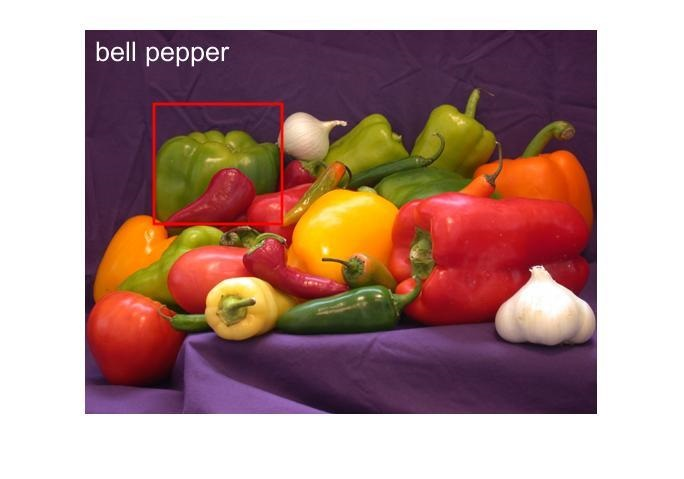bell pepper是甜椒的意思，我们发现效果还是不错的，感兴趣的同学可以多找几张测试图片试一下。用

 net.Layers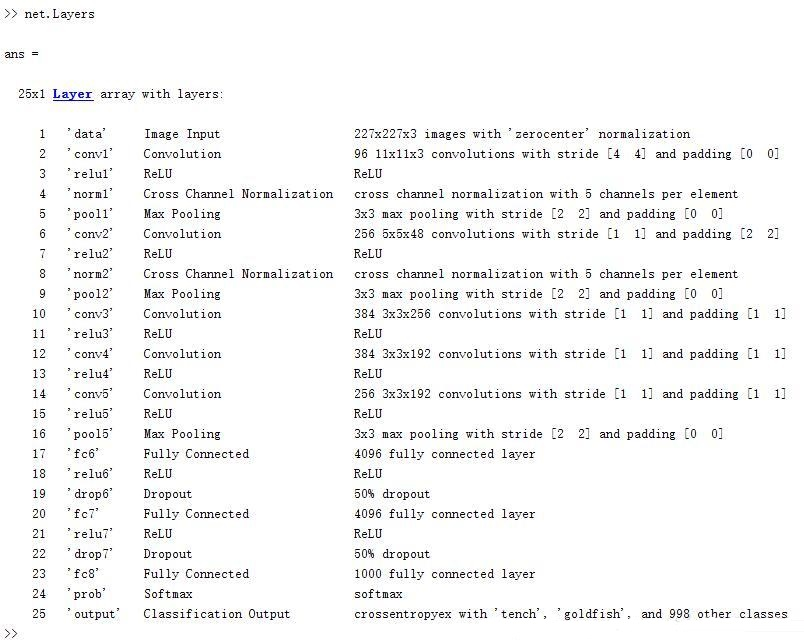net = vgg19; cropedim=imresize(cropedim,[224 224]);

3.2 迁移学习(transfer learning and fine-tune)

 %% transfer learning %读取训练集和测试集 digitDatasetPath = fullfile(matlabroot,'toolbox','nnet','nndemos', ... 'nndatasets','DigitDataset'); digitData = imageDatastore(digitDatasetPath, ... 'IncludeSubfolders',true,'LabelSource','foldernames'); [trainDigitData,testDigitData] = splitEachLabel(digitData,0.5,'randomize'); %显示前20个训练照片 numImages = numel(trainDigitData.Files); idx = randperm(numImages,20); for i = 1:20 subplot(4,5,i) I = readimage(trainDigitData, idx(i)); imshow(I) end % 获取matlab自己训练好的网络 load(fullfile(matlabroot,'examples','nnet','LettersClassificationNet.mat')) % 改变输出层的类别个数 layersTransfer = net.Layers(1:end-3); % 显示新的类别个数 numClasses = numel(categories(trainDigitData.Labels)); % 把最后三层替换成新的类别 layers = [... layersTransfer fullyConnectedLayer(numClasses,'WeightLearnRateFactor',20,'BiasLearnRateFactor',20) softmaxLayer classificationLayer]; optionsTransfer = trainingOptions('sgdm',... 'MaxEpochs',5,... 'InitialLearnRate',0.0001,... 'ExecutionEnvironment','cpu'); % 训练网络 netTransfer = trainNetwork(trainDigitData,layers,optionsTransfer); % 显示测试准确率 YPred = classify(netTransfer,testDigitData); YTest = testDigitData.Labels; accuracy = sum(YPred==YTest)/numel(YTest); % 显示测试结果 idx = 501:500:5000; figure for i = 1:numel(idx) subplot(3,3,i) I = readimage(testDigitData, idx(i)); label = char(YTest(idx(i))); imshow(I) title(label) end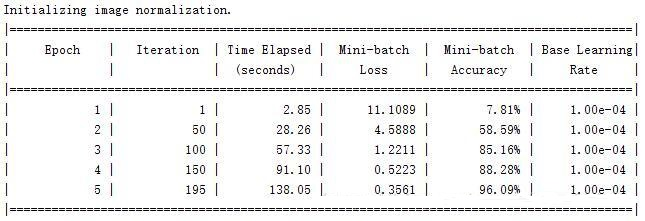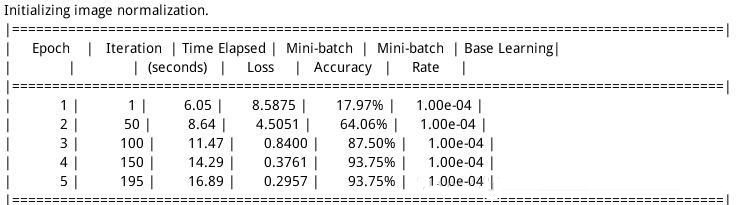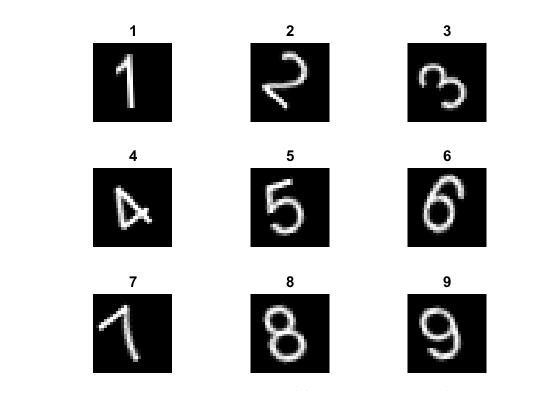3.3 分类问题(classification problem)

CNN之所以能引起广泛关注，就是在于它最初在图像分类方面取得很大的成功，后来人们发现对于其他的分类问题，CNN也有很好的性能。上边讲的迁移学习解决的也是一种分类问题，接下来的叙述也就建立在上文的基础上。

 layers = [ ... imageInputLayer([imsize imsize 1]) convolution2dLayer(5,150) reluLayer crossChannelNormalizationLayer(5,'Alpha',0.00005,'Beta',0.75,'K',1) %Norm layer1 convolution2dLayer(3,300,'Stride',1,'BiasLearnRateFactor',2) %Cov2 layer reluLayer fullyConnectedLayer(1) softmaxLayer classificationLayer];

 options = trainingOptions('sgdm', ... 'MaxEpochs',15, ... 'InitialLearnRate',1e-4, ... 'MiniBatchSize',256,... 'ExecutionEnvironment','gpu'); 'OutputFcn',functions);

 net = trainNetwork(trainData,layers,options);

 %读取数据集并保存成imageDatastore形式 digitDatasetPath = fullfile(matlabroot,'toolbox','nnet','nndemos',... 'nndatasets','DigitDataset'); digitData = imageDatastore(digitDatasetPath,... 'IncludeSubfolders',true,'LabelSource','foldernames'); %随机显示二十个训练集中的图片 figure; perm = randperm(10000,20); for i = 1:20 subplot(4,5,i); imshow(digitData.Files{perm(i)}); end %把数据集划分成训练集和测试集 trainingNumFiles = 750; rng(1) % For reproducibility [trainDigitData,testDigitData] = splitEachLabel(digitData,... trainingNumFiles,'randomize'); %建立自己的网络 layers = [imageInputLayer([28 28 1]); convolution2dLayer(5,20); reluLayer(); maxPooling2dLayer(2,'Stride',2); fullyConnectedLayer(10); softmaxLayer(); classificationLayer()]; %设定训练参数 options = trainingOptions('sgdm','MaxEpochs',20,... 'InitialLearnRate',0.0001); %训练网络 convnet = trainNetwork(trainDigitData,layers,options); %测试网络 YTest = classify(convnet,testDigitData); TTest = testDigitData.Labels; accuracy = sum(YTest == TTest)/numel(TTest); disp(accuracy);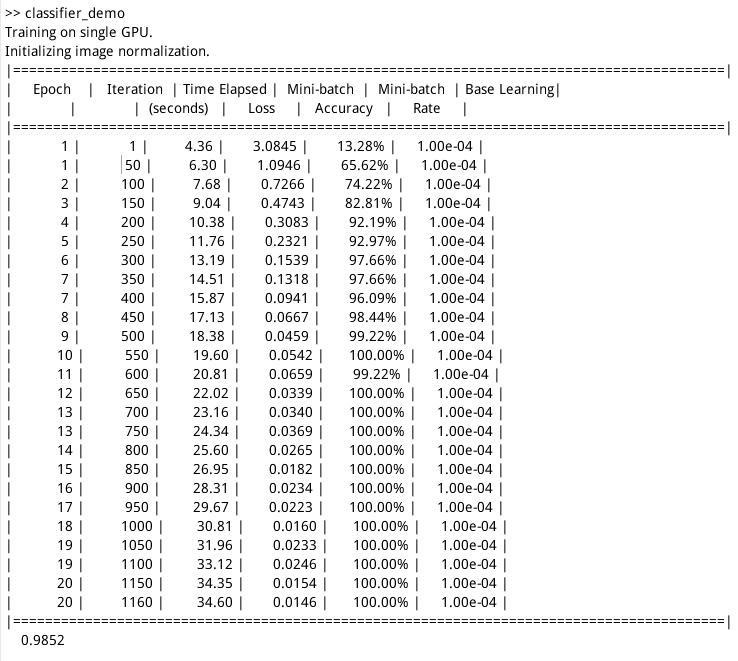3.4 回归问题(regression problem)

 %读取数据集 [trainImages,~,trainAngles] = digitTrain4DArrayData; %显示任意二十个结果 numTrainImages = size(trainImages,4); figure idx = randperm(numTrainImages,20); for i = 1:numel(idx) subplot(4,5,i) imshow(trainImages(:,:,:,idx(i))) drawnow end %建立回归网络 layers = [ ... imageInputLayer([28 28 1]) convolution2dLayer(12,25) reluLayer fullyConnectedLayer(1) regressionLayer]; %设置训练参数 functions={... @plotTrainingRMSE,... @(info)stopTrainingAtThreshold(info,0)}; options = trainingOptions('sgdm', ... 'MaxEpochs',20, ... 'InitialLearnRate',1e-3, ... 'MiniBatchSize',128,... 'ExecutionEnvironment','gpu',... 'OutputFcn',functions); %训练网络 net = trainNetwork(trainImages,trainAngles,layers,options); %测试网络 [testImages,~,testAngles] = digitTest4DArrayData; predictedTestAngles = predict(net,testImages); %查看拟合误差 predictionError = testAngles - predictedTestAngles; thr = 10; numCorrect = sum(abs(predictionError) < thr); numTestImages = size(testImages,4); accuracy = numCorrect/numTestImages; disp('accuracy'); disp(accuracy); squares = predictionError.^2; rmse = sqrt(mean(squares)); disp('the rmse'); disp(rmse); %train function function plotTrainingRMSE(info) persistent plotObj if info.State == "start" figure; plotObj = animatedline; xlabel("Iteration") ylabel("Training RMSE") elseif info.State == "iteration" addpoints(plotObj,info.Iteration,double(info.TrainingRMSE)) drawnow limitrate nocallbacks end end function stop = stopTrainingAtThreshold(info,thr) stop = false; if info.State ~= "iteration" return end persistent TrainingRMSE % Append accuracy for this iteration T= info.TrainingRMSE; % Evaluate mean of iteration accuracy and remove oldest entry stop = T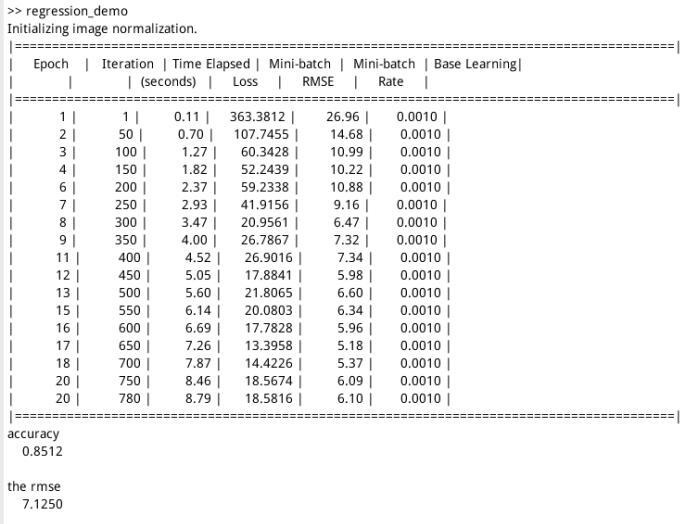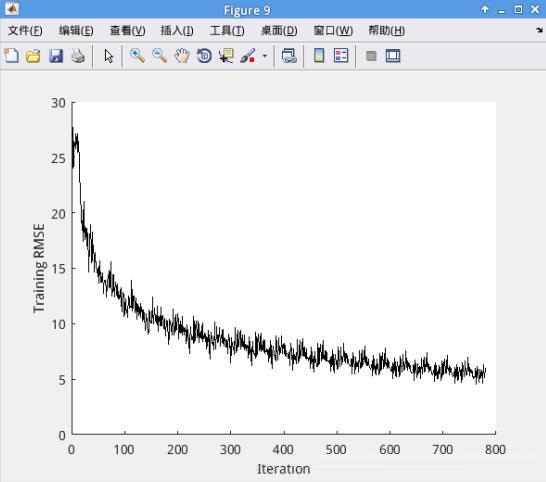3.3 检测问题(Detection problem)

1) 读取数据

data是个结构体类型的数据，主要是用来四个属性分别是detector, layers, result, vehicleTraining.

2) 抽取用于训练的图像

 trainingData = data.vehicleTrainingData; trainingData.imageFilename=fullfile(toolboxdir('vision'),'visiondata',... trainingData.imageFilename);

3) 读取网络结构

 layers=data.layers;

4) 设置训练选项

 options = trainingOptions('sgdm', ... 'InitialLearnRate',1e-6,... 'MaxEpochs',1,... 'ExecutionEnvironment','gpu',... 'CheckpointPath',tempdir);

5) 训练网络

 detector = trainFasterRCNNObjectDetector(trainingData,layers,options);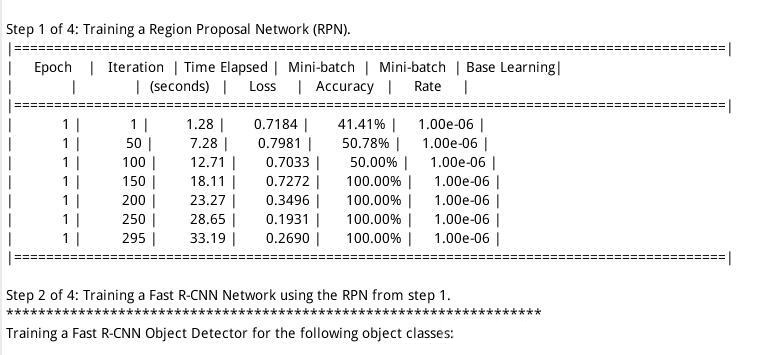6) 结果检测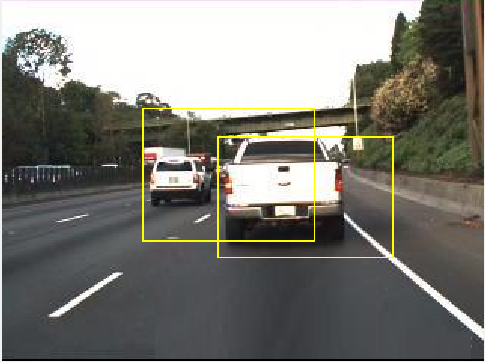1477 次浏览  评价: 好 中 差订阅捐助
 相关文章 我们该如何设计数据库 数据库设计经验谈 数据库设计过程 数据库编程总结
 相关文档 数据库性能调优技巧 数据库性能调整 数据库性能优化讲座 数据库系统性能调优系列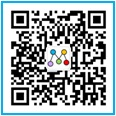每天2个文档/视频 扫描微信二维码订阅
 订阅技术月刊 获得每月300个技术资源